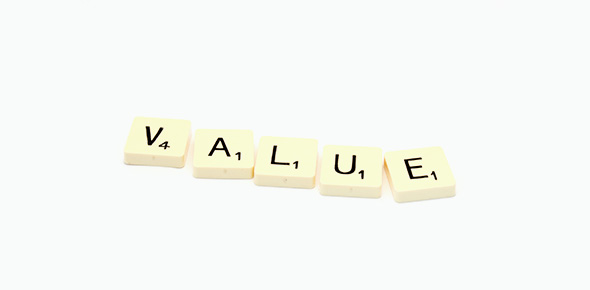# Place Value Of Whole Numbers

25 QuestionsSettingsThis quiz will assess your understanding of whole number place values. It will also assess your ability to correctly spell various words related to place value of whole numbers.

Related Topics
• 1.
What place value is the underlined digit in?        2,465
• A.

Tens

• B.

Ones

• C.

Hundreds

• D.

Thousands

• 2.
What place value is the underlined digit?         2,864
• A.

Hundreds

• B.

Tens

• C.

Ten-thousands

• D.

Thousands

• 3.
What place value is the underlined number?         573,968
• A.

Hundreds

• B.

Ones

• C.

Tenths

• D.

Tens

• 4.
What is the place value of the underlined number?         539,538
• A.

Hundreds

• B.

Millions

• C.

Ten thousands

• D.

Hundred thousands

• 5.
What is the correct spelling of the underlined place value 3,597?
• 6.
What is the place value of the underlined number?        389,462
• A.

Ten thousands

• B.

Hundreds

• C.

Thousands

• D.

Millions

• 7.
What is the standard form of this number?       500 + 30 +4
• A.

543

• B.

453

• C.

435

• D.

534

• 8.
What is the expanded form of this number?         918
• A.

900 + 18

• B.

900 + 8 + 1

• C.

900 + 10 + 8

• D.

90 + 10 + 8

• 9.
What is the correct spelling of the underlined place value       2,531,943
• 10.
What is the standard form of this number?          2000 + 700 + 90 + 5
• A.

2,795

• B.

2,975

• C.

2,597

• D.

2,759

• 11.
What is the expanded expanded of this number?         9,284
• A.

900 + 200 + 80 + 4

• B.

9000 + 200 + 80 + 4

• C.

9000 + 200 + 84

• D.

9000 + 20 + 80 +4

• 12.
Arrange these digits to make the biggest possible number.           8        1          9
• A.

819

• B.

918

• C.

891

• D.

981

• 13.
Arrange these digits to make the biggest possible number.            4        5       0      6
• A.

6,045

• B.

6,450

• C.

6,540

• D.

6,054

• 14.
Write this 2,856,204 in word form.
• 15.
Arrange these digits to make the smallest possible number:          957,412
• A.

142,579

• B.

124,579

• C.

124,759

• D.

124,795

• 16.
What is the correct spelling of 20?
• 17.
Write this number in words:          125
• 18.
What is the standard form of this number ?         Two thousand, one hundred, thirty-six
• A.

2,133

• B.

2,166

• C.

2,136

• D.

2,036

• 19.
What is the correct spelling of the underlined place value         732,496 ?
• 20.
What is the correct spelling of the underlined place value         4,392,857,216?
• 21.
• A.

Ones, Tens, Thousands, Millions, Billions, Trillions

• B.

Ones, Thousands, Millions, Billions, Trillions

• C.

Ones, Hundreds, Millions, Trillions, Billions

• D.

Ones, Thousands, Millions, Trillions, Billions

• 22.
There are only ten digits in our number system?
• A.

True

• B.

False

• 23.
What is the correct spelling of 30?
• 24.
What is the correct spelling of 50?
• 25.